Скачать презентацию Interest Rates and Bond Valuation T 1 Bond

d8e510f3be39e1dae27e40203a5d9e2b.ppt

• Количество слайдов: 23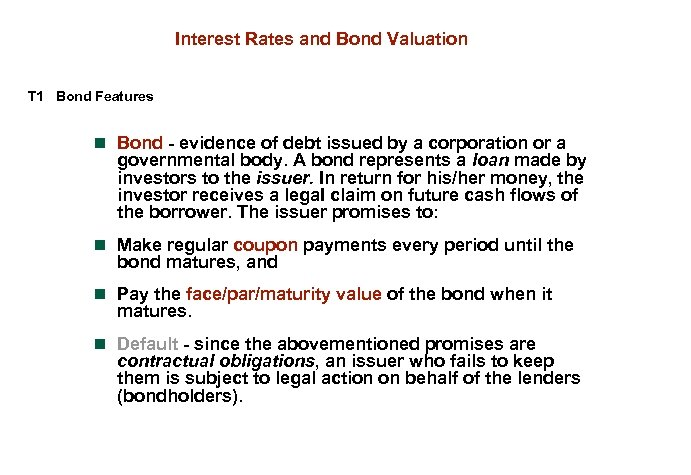Interest Rates and Bond Valuation T 1 Bond Features n Bond - evidence of debt issued by a corporation or a governmental body. A bond represents a loan made by investors to the issuer. In return for his/her money, the investor receives a lega. I claim on future cash flows of the borrower. The issuer promises to: n Make regular coupon payments every period until the bond matures, and n Pay the face/par/maturity value of the bond when it matures. n Default - since the abovementioned promises are contractual obligations, an issuer who fails to keep them is subject to legal action on behalf of the lenders (bondholders).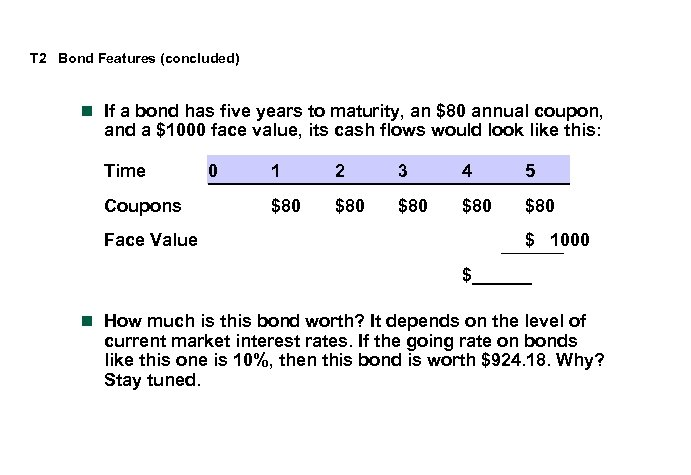T 2 Bond Features (concluded) n If a bond has five years to maturity, an \$80 annual coupon, and a \$1000 face value, its cash flows would look like this: Time Coupons Face Value 0 1 2 3 4 5 \$80 \$80 \$80 \$ 1000 \$______ n How much is this bond worth? It depends on the level of current market interest rates. If the going rate on bonds like this one is 10%, then this bond is worth \$924. 18. Why? Stay tuned.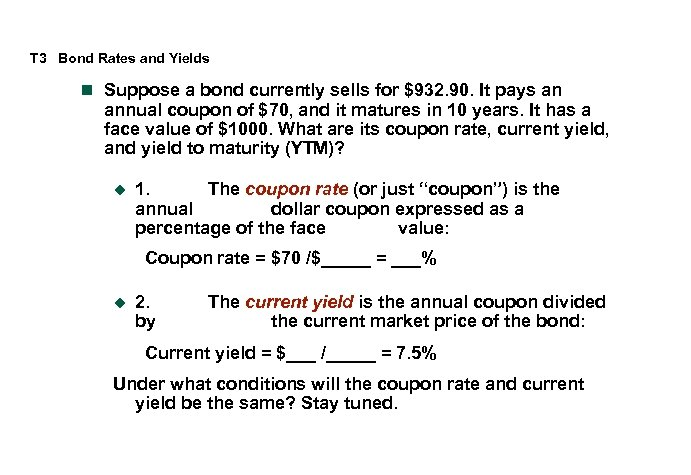T 3 Bond Rates and Yields n Suppose a bond currently sells for \$932. 90. It pays an annual coupon of \$70, and it matures in 10 years. It has a face value of \$1000. What are its coupon rate, current yield, and yield to maturity (YTM)? u 1. The coupon rate (or just “coupon”) is the annual dollar coupon expressed as a percentage of the face value: Coupon rate = \$70 /\$_____ = ___% u 2. by The current yield is the annual coupon divided the current market price of the bond: Current yield = \$___ /_____ = 7. 5% Under what conditions will the coupon rate and current yield be the same? Stay tuned.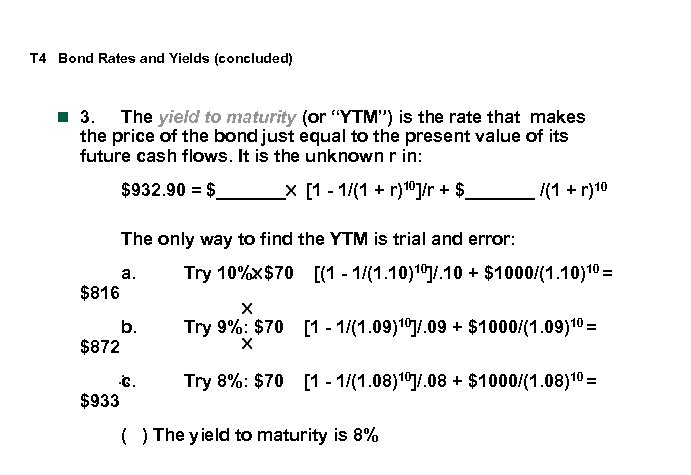T 4 Bond Rates and Yields (concluded) n 3. The yield to maturity (or “YTM”) is the rate that makes the price of the bond just equal to the present value of its future cash flows. It is the unknown r in: \$932. 90 = \$_______ [1 - 1/(1 + r)10]/r + \$_______ /(1 + r)10 The only way to find the YTM is trial and error: \$816 \$872 \$933 [(1 - 1/(1. 10)10]/. 10 + \$1000/(1. 10)10 = a. Try 10%: \$70 b. Try 9%: \$70 [1 - 1/(1. 09)10]/. 09 + \$1000/(1. 09)10 = c. Try 8%: \$70 [1 - 1/(1. 08)10]/. 08 + \$1000/(1. 08)10 = ( ) The yield to maturity is 8%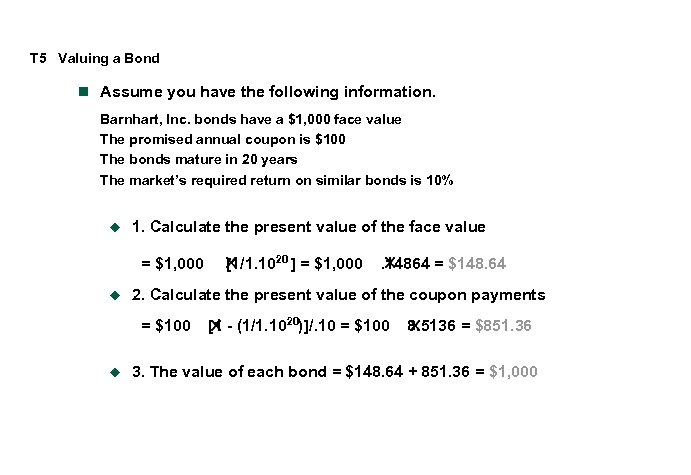T 5 Valuing a Bond n Assume you have the following information. Barnhart, Inc. bonds have a \$1, 000 face value The promised annual coupon is \$100 The bonds mature in 20 years The market’s required return on similar bonds is 10% u 1. Calculate the present value of the face value = \$1, 000 u . 14864 = \$148. 64 2. Calculate the present value of the coupon payments = \$100 u [1/1. 1020 ] = \$1, 000 [1 - (1/1. 1020)]/. 10 = \$100 8. 5136 = \$851. 36 3. The value of each bond = \$148. 64 + 851. 36 = \$1, 000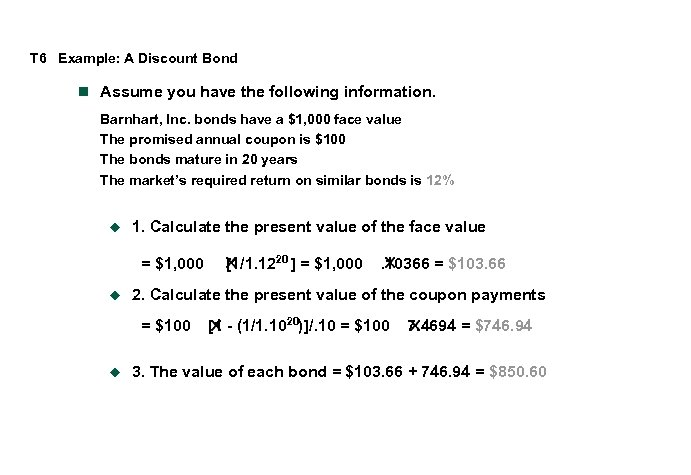T 6 Example: A Discount Bond n Assume you have the following information. Barnhart, Inc. bonds have a \$1, 000 face value The promised annual coupon is \$100 The bonds mature in 20 years The market’s required return on similar bonds is 12% u 1. Calculate the present value of the face value = \$1, 000 u . 10366 = \$103. 66 2. Calculate the present value of the coupon payments = \$100 u [1/1. 1220 ] = \$1, 000 [1 - (1/1. 1020)]/. 10 = \$100 7. 4694 = \$746. 94 3. The value of each bond = \$103. 66 + 746. 94 = \$850. 60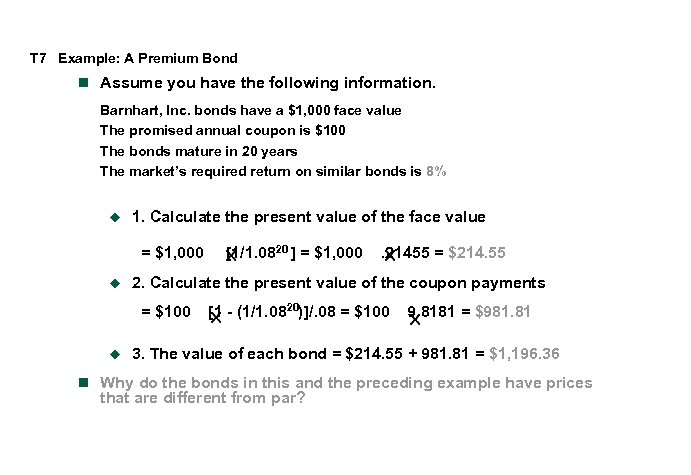T 7 Example: A Premium Bond n Assume you have the following information. Barnhart, Inc. bonds have a \$1, 000 face value The promised annual coupon is \$100 The bonds mature in 20 years The market’s required return on similar bonds is 8% u 1. Calculate the present value of the face value = \$1, 000 u . 21455 = \$214. 55 2. Calculate the present value of the coupon payments = \$100 u [1/1. 0820 ] = \$1, 000 [1 - (1/1. 0820)]/. 08 = \$100 9. 8181 = \$981. 81 3. The value of each bond = \$214. 55 + 981. 81 = \$1, 196. 36 n Why do the bonds in this and the preceding example have prices that are different from par?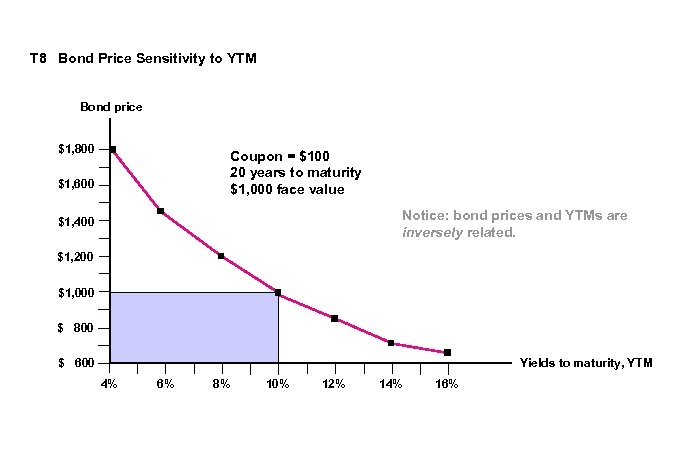T 8 Bond Price Sensitivity to YTM Bond price \$1, 800 Coupon = \$100 20 years to maturity \$1, 000 face value \$1, 600 Notice: bond prices and YTMs are inversely related. \$1, 400 \$1, 200 \$1, 000 \$ 800 \$ 600 Yields to maturity, YTM 4% 6% 8% 10% 12% 14% 16%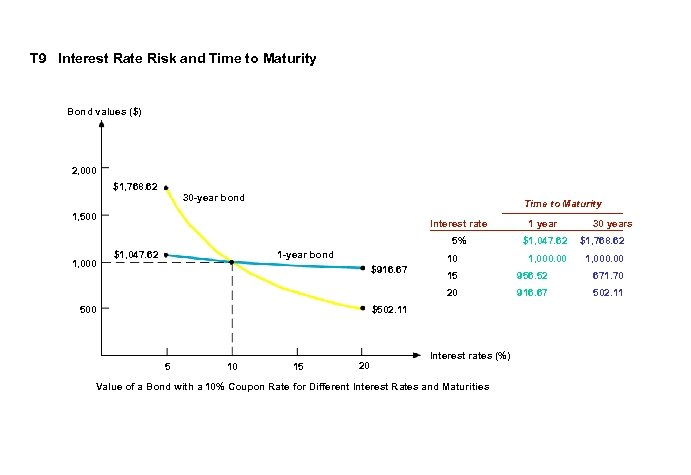T 9 Interest Rate Risk and Time to Maturity Bond values (\$) 2, 000 \$1, 768. 62 30 -year bond Time to Maturity 1, 500 \$1, 047. 62 1 -year bond 1 year 5% 1, 000 Interest rate \$1, 047. 62 \$1, 768. 62 1, 000. 00 10 \$916. 67 30 years 956. 52 671. 70 20 500 15 916. 67 502. 11 \$502. 11 5 10 15 20 Interest rates (%) Value of a Bond with a 10% Coupon Rate for Different Interest Rates and Maturities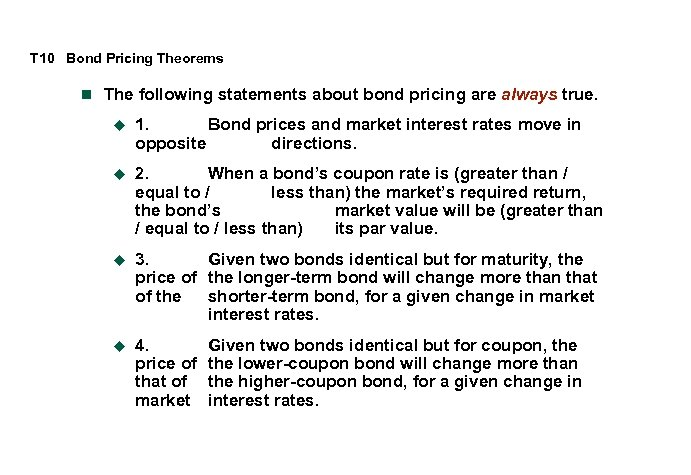T 10 Bond Pricing Theorems n The following statements about bond pricing are always true. u 1. Bond prices and market interest rates move in opposite directions. u 2. When a bond’s coupon rate is (greater than / equal to / less than) the market’s required return, the bond’s market value will be (greater than / equal to / less than) its par value. u 3. Given two bonds identical but for maturity, the price of the longer-term bond will change more than that of the shorter-term bond, for a given change in market interest rates. u 4. price of that of market Given two bonds identical but for coupon, the lower-coupon bond will change more than the higher-coupon bond, for a given change in interest rates.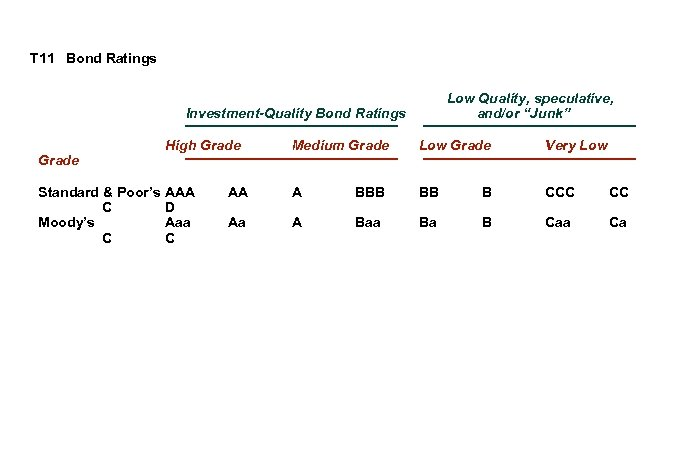T 11 Bond Ratings Low Quality, speculative, and/or “Junk” Investment-Quality Bond Ratings Grade Standard & Poor’s C Moody’s C High Grade Medium Grade Low Grade Very Low AAA D Aaa C AA A BBB BB B CCC CC Aa A Baa Ba B Caa Ca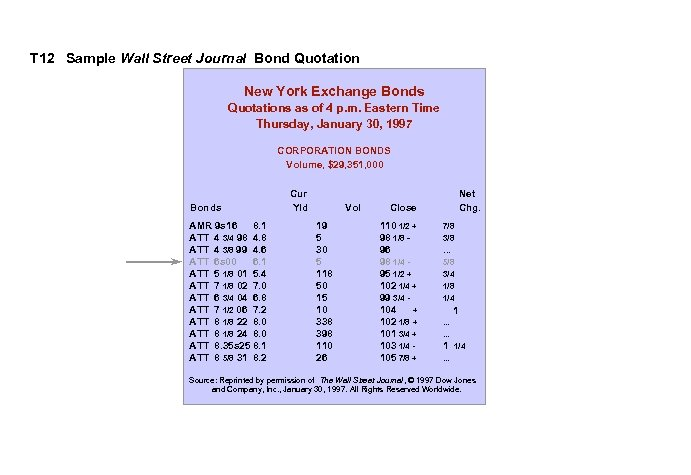T 12 Sample Wall Street Journal Bond Quotation New York Exchange Bonds Quotations as of 4 p. m. Eastern Time Thursday, January 30, 1997 CORPORATION BONDS Volume, \$29, 351, 000 Bonds AMR 9 s 16 8. 1 ATT 4 3/4 98 4. 8 ATT 4 3/8 99 4. 6 ATT 6 s 00 6. 1 ATT 5 1/8 01 5. 4 ATT 7 1/8 02 7. 0 ATT 6 3/4 04 6. 8 ATT 7 1/2 06 7. 2 ATT 8 1/8 22 8. 0 ATT 8 1/8 24 8. 0 ATT 8. 35 s 25 8. 1 ATT 8 5/8 31 8. 2 Cur Yld Vol 19 5 30 5 118 50 15 10 338 398 110 26 Net Chg. Close 110 1/2 + 98 1/8 96 98 1/4 95 1/2 + 102 1/4 + 99 3/4 104 + 102 1/8 + 101 3/4 + 103 1/4 105 7/8 + 7/8 3/8 . . . 5/8 3/4 1/8 1/4 1. . . 1 1/4 . . . Source: Reprinted by permission of The Wall Street Journal, © 1997 Dow Jones and Company, Inc. , January 30, 1997. All Rights Reserved Worldwide.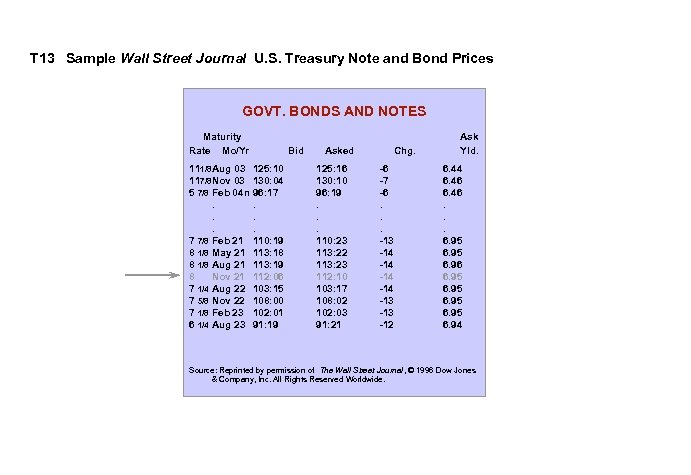T 13 Sample Wall Street Journal U. S. Treasury Note and Bond Prices GOVT. BONDS AND NOTES Maturity Rate Mo/Yr 111/8 Aug 03 117/8 Nov 03 5 7/8 Feb 04 n. . . 7 7/8 Feb 21 8 1/8 May 21 8 1/8 Aug 21 8 Nov 21 7 1/4 Aug 22 7 5/8 Nov 22 7 1/8 Feb 23 6 1/4 Aug 23 Bid 125: 10 130: 04 96: 17. . . 110: 19 113: 18 113: 19 112: 06 103: 15 108: 00 102: 01 91: 19 Asked 125: 16 130: 10 96: 19. . . 110: 23 113: 22 113: 23 112: 10 103: 17 108: 02 102: 03 91: 21 Chg. -6 -7 -6. . . -13 -14 -14 -13 -12 Ask Yld. 6. 44 6. 46. . . 6. 95 6. 96 6. 95 6. 94 Source: Reprinted by permission of The Wall Street Journal, © 1996 Dow Jones & Company, Inc. All Rights Reserved Worldwide.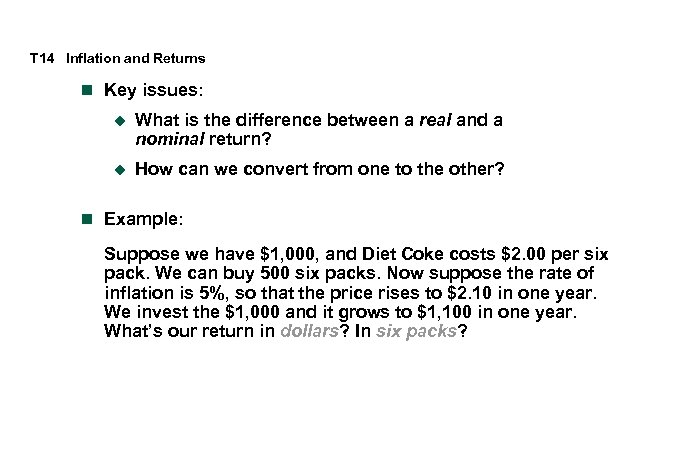T 14 Inflation and Returns n Key issues: u What is the difference between a real and a nominal return? u How can we convert from one to the other? n Example: Suppose we have \$1, 000, and Diet Coke costs \$2. 00 per six pack. We can buy 500 six packs. Now suppose the rate of inflation is 5%, so that the price rises to \$2. 10 in one year. We invest the \$1, 000 and it grows to \$1, 100 in one year. What’s our return in dollars? In six packs?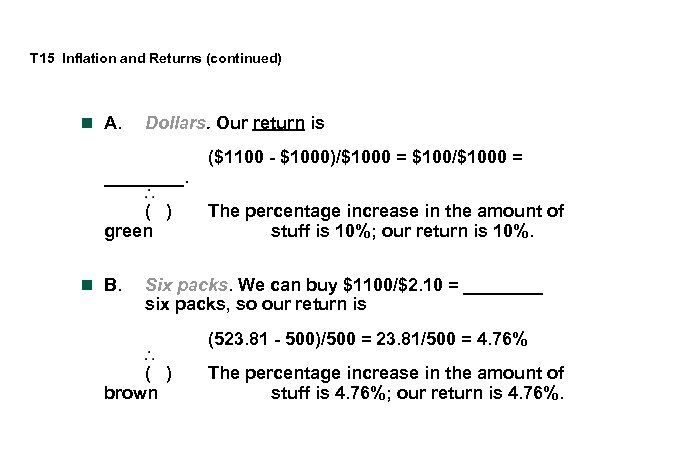T 15 Inflation and Returns (continued) n A. Dollars. Our return is ____. ( ) green n B. (\$1100 - \$1000)/\$1000 = \$100/\$1000 = The percentage increase in the amount of stuff is 10%; our return is 10%. Six packs. We can buy \$1100/\$2. 10 = ____ six packs, so our return is (523. 81 - 500)/500 = 23. 81/500 = 4. 76% ( ) brown The percentage increase in the amount of stuff is 4. 76%; our return is 4. 76%.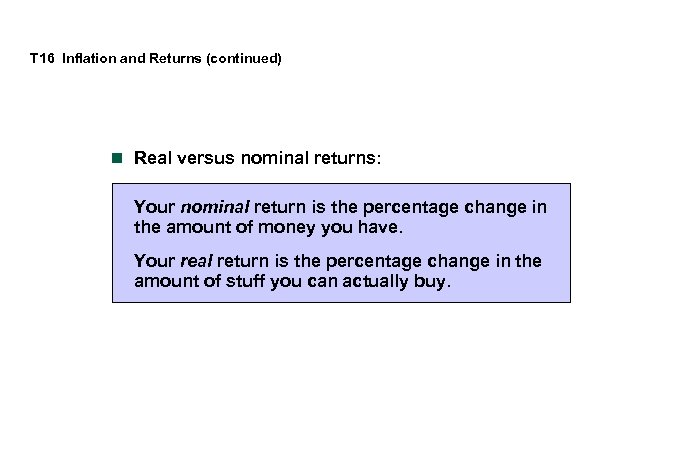T 16 Inflation and Returns (continued) n Real versus nominal returns: Your nominal return is the percentage change in the amount of money you have. Your real return is the percentage change in the amount of stuff you can actually buy.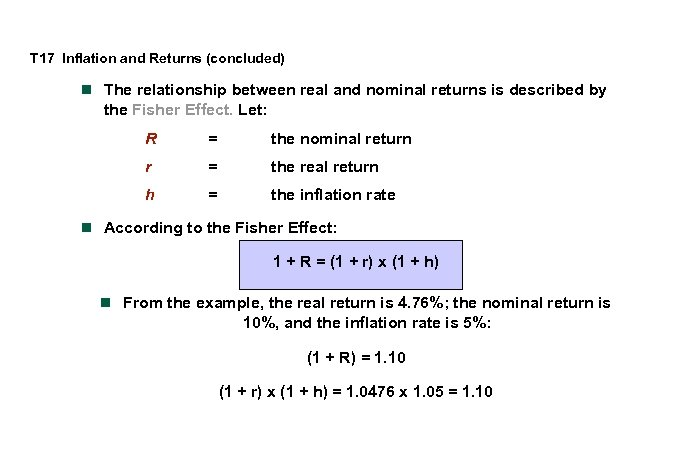T 17 Inflation and Returns (concluded) n The relationship between real and nominal returns is described by the Fisher Effect. Let: R = the nominal return r = the real return h = the inflation rate n According to the Fisher Effect: 1 + R = (1 + r) x (1 + h) n From the example, the real return is 4. 76%; the nominal return is 10%, and the inflation rate is 5%: (1 + R) = 1. 10 (1 + r) x (1 + h) = 1. 0476 x 1. 05 = 1. 10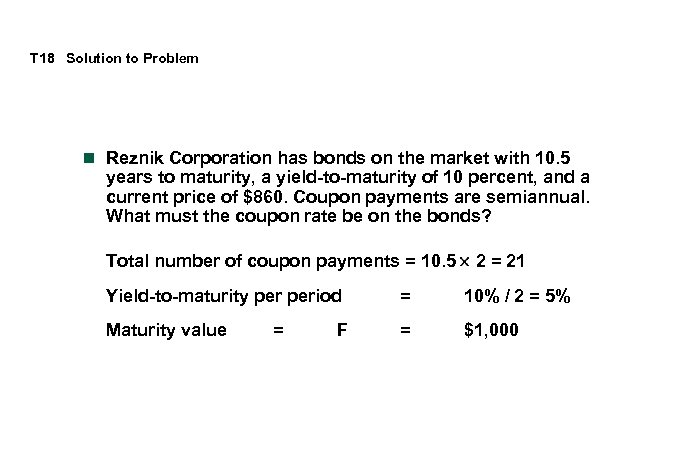T 18 Solution to Problem n Reznik Corporation has bonds on the market with 10. 5 years to maturity, a yield-to-maturity of 10 percent, and a current price of \$860. Coupon payments are semiannual. What must the coupon rate be on the bonds? Total number of coupon payments = 10. 5 2 = 21 Yield-to-maturity period = 10% / 2 = 5% Maturity value = \$1, 000 = F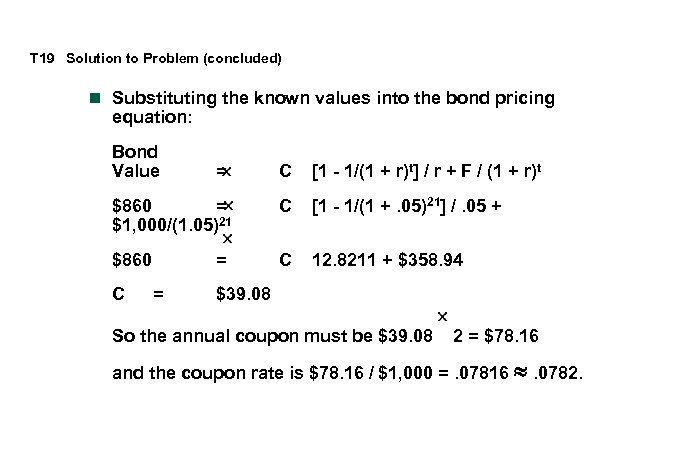T 19 Solution to Problem (concluded) n Substituting the known values into the bond pricing equation: Bond Value C [1 - 1/(1 + r)t] / r + F / (1 + r)t \$860 = \$1, 000/(1. 05)21 C [1 - 1/(1 +. 05)21] /. 05 + \$860 C 12. 8211 + \$358. 94 C = = = \$39. 08 So the annual coupon must be \$39. 08 2 = \$78. 16 and the coupon rate is \$78. 16 / \$1, 000 =. 07816 » . 0782.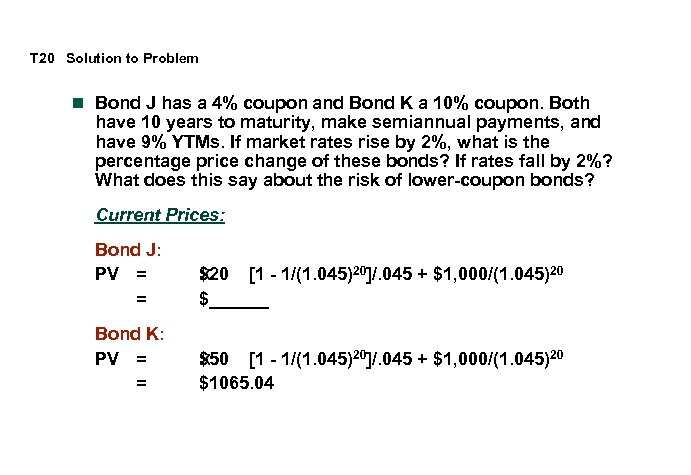T 20 Solution to Problem n Bond J has a 4% coupon and Bond K a 10% coupon. Both have 10 years to maturity, make semiannual payments, and have 9% YTMs. If market rates rise by 2%, what is the percentage price change of these bonds? If rates fall by 2%? What does this say about the risk of lower-coupon bonds? Current Prices: Bond J: PV = = \$20 [1 - 1/(1. 045)20]/. 045 + \$1, 000/(1. 045)20 \$______ Bond K: PV = = \$50 [1 - 1/(1. 045)20]/. 045 + \$1, 000/(1. 045)20 \$1065. 04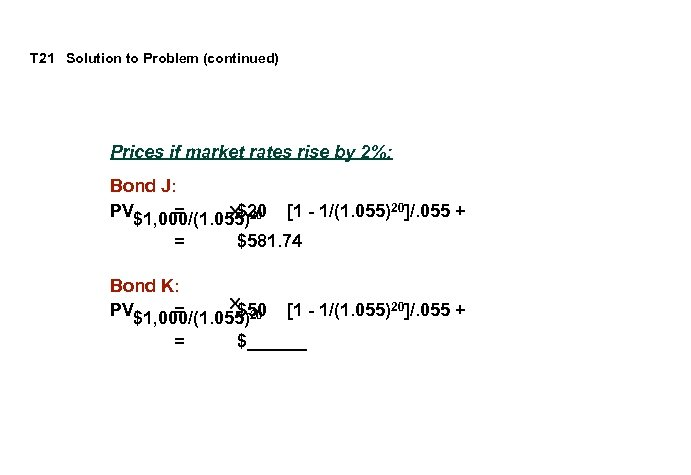T 21 Solution to Problem (continued) Prices if market rates rise by 2%: Bond J: PV\$1, 000/(1. 055)20 = \$581. 74 Bond K: PV\$1, 000/(1. 055)20 = \$50 = [1 - 1/(1. 055)20]/. 055 + \$______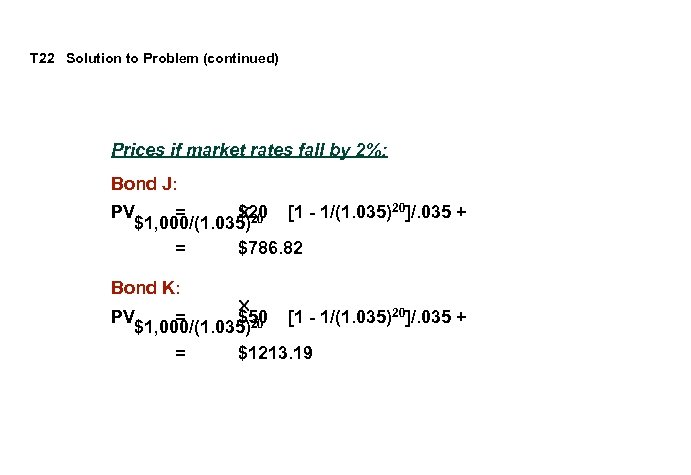T 22 Solution to Problem (continued) Prices if market rates fall by 2%: Bond J: PV = \$20 [1 - 1/(1. 035)20]/. 035 + \$1, 000/(1. 035)20 = \$786. 82 Bond K: PV = \$50 [1 - 1/(1. 035)20]/. 035 + \$1, 000/(1. 035)20 = \$1213. 19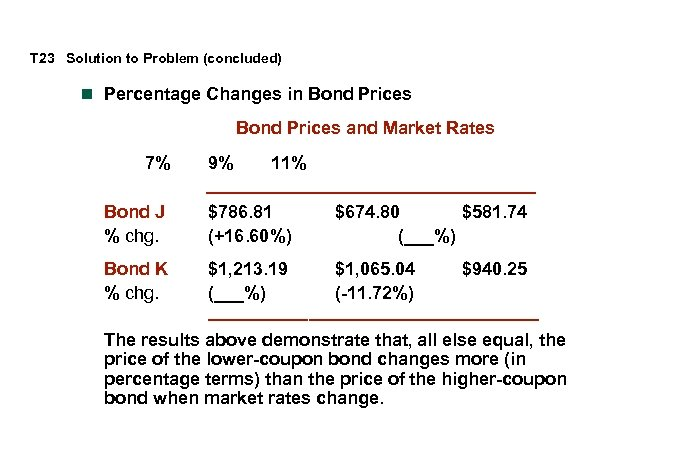T 23 Solution to Problem (concluded) n Percentage Changes in Bond Prices and Market Rates 7% 9% 11% _________________ Bond J % chg. \$786. 81 (+16. 60%) \$674. 80 \$581. 74 (___%) Bond K % chg. \$1, 213. 19 \$1, 065. 04 \$940. 25 (___%) (-11. 72%) _________________ The results above demonstrate that, all else equal, the price of the lower-coupon bond changes more (in percentage terms) than the price of the higher-coupon bond when market rates change.